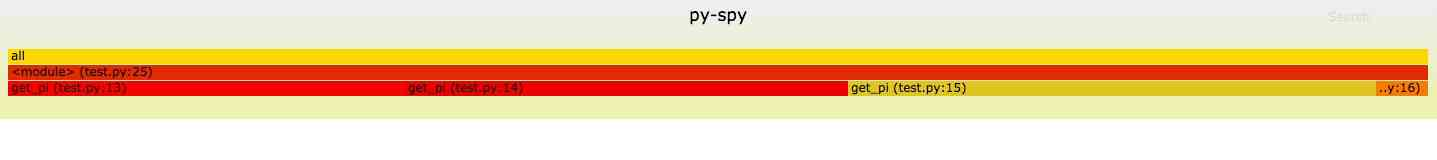# 用py-spy分析Python代码

``````pip install py-spy
``````

``````from __future__ import division

def get_pi(number):
number1 = number+10
b = 10**number1
x1 = b*4//5
x2 = b// -239
he = x1+x2
number *= 2

for i in range(3, number,2):
x1 //= -25
x2 //= -57121
x = (x1+x2) // i
he += x

pai = he*4
pai //= 10**10
paistring=str(pai)
result = paistring+str('.')+paistring[1:len(paistring)]
print(result)

get_pi(1000000)
``````

## 1. dump py-spy dump

``````[root@pyhost ~]# py-spy dump --pid 21512
Process 21512: python test.py
Python v2.7.5 (/usr/bin/python2.7)

get_pi (test.py:15)
<module> (test.py:25)
``````

## 2. top

top 命令可以展示哪些函数被调用执行的次数最多，由于我测试的程序只有一个函数，因此只显示了一个函数。

``````[root@pyhost ~]# py-spy top --pid 22135

Collecting samples from 'python test.py' (python v2.7.5)
Total Samples 800
GIL: 100.00%, Active: 100.00%, Threads: 1

%Own   %Total  OwnTime  TotalTime  Function (filename:line)
36.00%  36.00%    3.06s     3.06s   get_pi (test.py:15)
18.00%  18.00%    2.38s     2.38s   get_pi (test.py:14)
40.00%  40.00%    2.32s     2.32s   get_pi (test.py:13)
6.00%   6.00%   0.240s    0.240s   get_pi (test.py:16)
0.00% 100.00%   0.000s     8.00s   <module> (test.py:25)
``````

## 3. 火焰图

``````py-spy record -o profile.svg --pid 21512
``````QQ交流群: 211426309